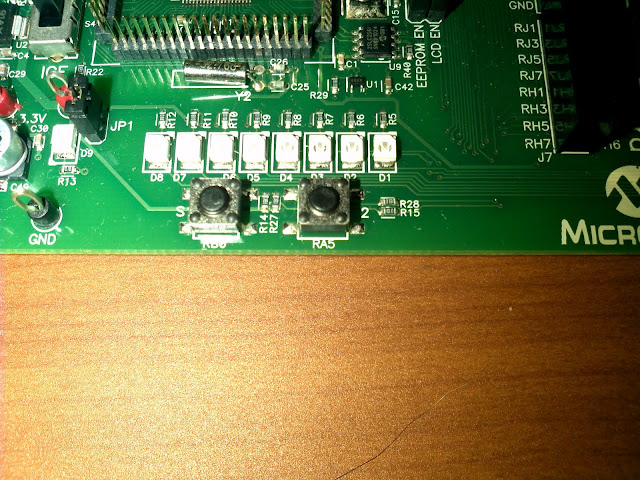# PIC18 Explorer Board Tutorial: How to Use The Buttons In Your Board RA5 and RB0

by on June 2, 2011Buttons RA5 and RB0 waiting to be pushed

In a previous tutorial I showed you how to use the button in pin RB0 to turn on an LED connected to a pin. In this tutorial I will show you how to use both buttons (RB0 and RA5) to create a “moving” light in the eight lights connected to port D.

Let’s take a look at what’s new instead of what’s old. From myprevious tutorials on the board you already know how button input works and how the lights in port D work.

## Bit Shifting:

Bit shifting is the process of moving the bits in a number either to the left or right, arithmetically moving a shift to the left is equal to multiplying the number times two however many times you shift it, moving it the rights is diving by two however many times you shift.

### Example:

```0b00001<<3; // or 1<<3 , where 1 is the number we shift and 3 is the number of shifts
```

would result in

```0b01000; // or 8 which is equal to 1*2*2*2 ( times two for every time we shift)
```

If you want to shift to the right use >> instead of <<

## The Program

Let's make program that's let's use move a light to the right or left depending on which button we press. Let's start off with having the right most light turned on.

```#include <p18f8722.h>
#include <delays.h>

#pragma config OSC=HS // high speed oscillator
#pragma config WDT=OFF // watch dog off

void main()
{
TRISD=0x00; // port D as output
TRISB=0xff; // PORT B as input
TRISA=0xff; // PORT A as input

MEMCONbits.EBDIS=1; // enable I/O functions of port D (where the lights are)

}

```

Now if we press the button on the left (RB0) the light will move left and pressing RA5 will make the light move right.

```while(1)
{
// RB0 button code
if(PORTBbits.RB0==0) // if RB0 is pressed
{
Delay10KTCYx(50); // wait
if(PORTD==0b10000000) // if light is all the way to the left
PORTD=PORTD; // don't move light
else // if it's not all the way to the left
PORTD=PORTD<<1; // move it to the left once
}

// RA5 button code
if(PORTAbits.RA5==0) // if RA5 is pressed
{
Delay10KTCYx(50); // wait
if(PORTD==0b00000001) // if light is all the way to the right
PORTD=PORTD; // don't move light
else // if light is not all the way to the light
PORTD=PORTD>>1; // move the to the right once
}
}
```

## How To Make Port A Digital

The program above does not work because port A is by default, to make port A digital (according to the datasheet) we have to enable the ADCON1 bits.

``` /*
*/

ADCON1=0b00001111; // enable input port A, equivalent to the 4 lines above

```

## The Final Program

```#include <p18f8722.h>
#include <delays.h>

#pragma config OSC=HS // high speed oscillator
#pragma config WDT=OFF // watch dog off

void main()
{
TRISD=0x00; // port D as output
TRISB=0xff; // PORT B as input
TRISA=0xff; // PORT A as input

MEMCONbits.EBDIS=1; // enable I/O functions of port D (where the lights are)

/*
*/

ADCON1=0b00001111; // enable input port A, equivalent to the 4 lines above

while(1)
{
// RB0 button code
if(PORTBbits.RB0==0) // if RB0 is pressed
{
Delay10KTCYx(50); // wait
if(PORTD==0b10000000) // if light is all the way to the left
PORTD=PORTD; // don't move light
else // if it's not all the way to the left
PORTD=PORTD<<1; // move it to the left once
}

// RA5 button code
if(PORTAbits.RA5==0) // if RA5 is pressed
{
Delay10KTCYx(50); // wait
if(PORTD==0b00000001) // if light is all the way to the right
PORTD=PORTD; // don't move light
else // if light is not all the way to the light
PORTD=PORTD>>1; // move the to the right once
}
}
}
```

If you prefer to watch rather than read here is the video, the result is also shown at the end of the video